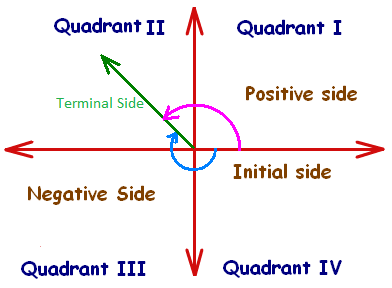Click to Chat

1800-1023-196

+91-120-4616500

CART 0

• 0

MY CART (5)

Use Coupon: CART20 and get 20% off on all online Study Material

ITEM
DETAILS
MRP
DISCOUNT
FINAL PRICE
Total Price: Rs.

There are no items in this cart.
Continue Shopping```Multiple and Sub Multiple AnglesAngle is the pivot around which the topic of Trigonometry revolves. Trigonometry studies angles and their relationship. When there is a single function or a single angle, the computation is comparatively easy. But there are various formulae for multiples and sub multiples of angles too. These multiple and sub multiple angles formula should rather be called as identities as they hold true for all angles. These formulae prove useful in solving intricate trigonometric equations.

It is also possible to find the trigonometric ratios of negative angles, multiple and sub multiple of an angle or compound angles. The coming sections illustrate trigonometric ratios of multiple and sub multiple angles along with various examples. Drafted below are the various topics covered under this head in the following sections:

Trigonometric Ratios of Compound Angles

Trigonometric Ratios of Submultiple of an angle

Trigonometric Ratios for Negative Angles

Solved examples on Trigonometric Ratio

We shall just give an outline of these topics here. Those interested in going into the intricacies of the topics can refer the following sections.

Compound Angles

Angles composed of an algebraic sum or difference of two or more angles are called compound angles. Trigonometric ratios of compound angles include evaluation of trigonometric sum or trigonometric difference of two or more angles. Some of the trigonometric identities regarding the compound angles are listed below:

sin(A + B) = sinA cosB + cosA sinB

sin(A – B) = sinA cosB – cos A sin B

cos(A + B) = cosA cosB – sinA sinB

Submultiples of an Angle

As the name suggests, trigonometric ratio of a submultiple of an angle means when we try to find out some trigonometric value of an angle of the type A/2 or A/3.

Some of the trigonometric identities for the submultiple of an angle are:

| sin A/2 + cos A/2| = √(1 + sin A)

| sin A/2 - cos A/2| = √(1 - sin A)

tan A/2 = ±√(1 - cos A)/(1 + cos A)

Negative Angles

By convention, an angle measured in the anti-clockwise direction of positive x-axis is considered to be positive and hence, the angle measured in the clockwise direction is considered to be negative. Angle is an extremely important concept in trigonometry and the trigonometric results tend to change for negative angles.Multiple and sub multiple angles are an important topic of the IIT JEE syllabus. Multiple choice questions on multiple and sub multiple angles are often asked in various competitions. Students are advised to master these concepts in order to remain competitive in the JEE.

Related Resources:

Look into the Revision Notes on Trigonometry for a quick revision.

Various Recommended Books of Mathematics are just a click away.

To read more, Buy study materials of Inverse Trigonometric Functions comprising study notes, revision notes, video lectures, previous year solved questions etc. Also browse for more study materials on Mathematics here.

```### Course Features

• 731 Video Lectures
• Revision Notes
• Previous Year Papers
• Mind Map
• Study Planner
• NCERT Solutions
• Discussion Forum
• Test paper with Video Solution